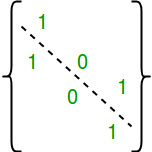# Number of Symmetric Relations on a Set

Given a number n, find out number of Symmetric Relations on a set of first n natural numbers {1, 2, ..n}.

Examples:

```Input  : n = 2
Output : 8
Given set is {1, 2}. Below are all symmetric relation.
{}
{(1, 1)},
{(2, 2)},
{(1, 1), (2, 2)},
{(1, 2), (2, 1)}
{(1, 1), (1, 2), (2, 1)},
{(2, 2), (1, 2), (2, 1)},
{(1, 1), (1, 2), (2, 1), (1, 2)}

Input  : n = 3
Output : 64
```

## Recommended: Please try your approach on {IDE} first, before moving on to the solution.

A Relation ‘R’ on Set A is said be Symmetric if xRy then yRx for every x, y ∈ A
or if (x, y) ∈ R, then (y, x) ∈ R for every x, y?A

Total number of symmetric relations is 2n(n+1)/2.

How does this formula work?

A relation R is symmetric if the value of every cell (i, j) is same as that cell (j, i). The diagonals can have any value.There are n diagonal values, total possible combination of diagonal values = 2n
There are n2 – n non-diagonal values. We can only choose different value for half of them, because when we choose a value for cell (i, j), cell (j, i) gets same value.
So combination of non-diagonal values = 2(n2 – n)/2

Overall combination = 2n * 2(n2 – n)/2 = 2n(n+1)/2

## C++

 `// C++ program to count total symmetric relations ` `// on a set of natural numbers. ` `#include ` ` `  `// function find the square of n ` `unsigned ``int` `countSymmetric(unsigned ``int` `n) ` `{ ` `    ``// Base case ` `    ``if` `(n == 0) ` `        ``return` `1; ` ` `  `   ``// Return 2^(n(n + 1)/2) ` `   ``return` `1 << ((n * (n + 1))/2); ` `} ` ` `  `// Driver code ` `int` `main() ` `{ ` `    ``unsigned ``int` `n = 3; ` ` `  `    ``printf``(``"%u"``, countSymmetric(n)); ` `    ``return` `0; ` `} `

## Java

 `// Java program to count total symmetric  ` `// relations on a set of natural numbers. ` `import` `java.io.*; ` `import` `java.util.*; ` ` `  `class` `GFG { ` ` `  `    ``// function find the square of n ` `    ``static` `int` `countSymmetric(``int` `n) ` `    ``{ ` `        ``// Base case ` `        ``if` `(n == ``0``) ` `            ``return` `1``; ` `     `  `    ``// Return 2^(n(n + 1)/2) ` `    ``return` `1` `<< ((n * (n + ``1``)) / ``2``); ` `    ``} ` ` `  `    ``// Driver code ` `    ``public` `static` `void` `main (String[] args)  ` `    ``{ ` `        ``int` `n = ``3``; ` `        ``System.out.println(countSymmetric(n)); ` `    ``} ` `} ` ` `  ` `  `// This code is contributed by Nikita Tiwari. `

## Python3

 `# Python 3 program to count ` `# total symmetric relations ` `# on a set of natural numbers. ` ` `  `# function find the square of n ` `def` `countSymmetric(n) : ` `    ``# Base case ` `    ``if` `(n ``=``=` `0``) : ` `        ``return` `1` `  `  `    ``# Return 2^(n(n + 1)/2) ` `    ``return` `(``1` `<< ((n ``*` `(n ``+` `1``))``/``/``2``)) ` ` `  `  `  `# Driver code ` ` `  `n ``=` `3` `print``(countSymmetric(n)) ` ` `  `# This code is contributed ` `# by Nikita Tiwari. `

## C#

 `// C# program to count total symmetric  ` `// relations on a set of natural numbers. ` `using` `System; ` ` `  `class` `GFG { ` ` `  `    ``// function find the square of n ` `    ``static` `int` `countSymmetric(``int` `n) ` `    ``{ ` `        ``// Base case ` `        ``if` `(n == 0) ` `            ``return` `1; ` `     `  `    ``// Return 2^(n(n + 1)/2) ` `    ``return` `1 << ((n * (n + 1)) / 2); ` `    ``} ` ` `  `    ``// Driver code ` `    ``public` `static` `void` `Main ()  ` `    ``{ ` `        ``int` `n = 3; ` `        ``Console.WriteLine(countSymmetric(n)); ` `    ``} ` `} ` ` `  ` `  `// This code is contributed by vt_m. `

## PHP

 ` `

Output:

```64
```

Attention reader! Don’t stop learning now. Get hold of all the important DSA concepts with the DSA Self Paced Course at a student-friendly price and become industry ready.

My Personal Notes arrow_drop_upCheck out this Author's contributed articles.

If you like GeeksforGeeks and would like to contribute, you can also write an article using contribute.geeksforgeeks.org or mail your article to contribute@geeksforgeeks.org. See your article appearing on the GeeksforGeeks main page and help other Geeks.

Please Improve this article if you find anything incorrect by clicking on the "Improve Article" button below.

Improved By : vt_m